# Construct with ruler and compasses, angles of following measures:(a) $60^{\circ}$(b) $30^{\circ}$(c) $90^{\circ}$(d) $120^{\circ}$(e) $45^{\circ}$(f) $135^{\circ}$

To do:

We have to construct angles of given measures.

Solution:

(a)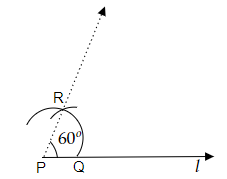Steps of construction:

(i) Let us draw a line $l$ of any length and mark a point $P$ on the line $l$.

(ii) Now, by taking compasses with any radius let us draw an arc from point $P$ and mark the point of intersection of the arc with the line $l$ as point $R$.

(iii) Now, by taking compasses with the same radius as before let us draw an arc on the previous arc from point $R$ and mark the point of intersection as $S$.

(iv) Now, let us join the points $P$ and $S$.Therefore, the required angle of measure $60^o$ with the line $l$ is formed.

(b)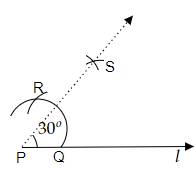Steps of construction:

(i) Let us draw a line $l$ of any length and mark a point $P$ on the line $l$. Now, by taking compasses with any radius let us draw an arc from point $P$ and mark the point of intersection of the arc with the line $l$ as $Q$.

(ii) Now, by taking compasses with the same radius as before let us draw another arc from point $Q$ and mark the point of intersection of this arc with the previous arc as $R$.

(iii) Now, by taking compasses with the length of radius greater than half of the length from point $Q$ to point $R$ let us draw arcs from point $P$ and $Q$ respectively and let us mark the point of intersection of this arcs to each other as point $S$.

(iv) Now, let us join the point $S$ and point $P$. Therefore, $\overline{PS}$ has the required measure of angle $30^o$ with the line $l$.

(c)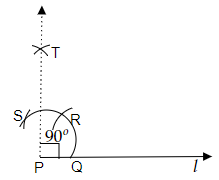Steps of construction:

(i) Let us draw a line $l$ of any length and mark a point $P$ on the line $l$. Now, by taking compasses with any radius let us draw an arc from point $P$ and mark the point of intersection of this arc with line $l$ as $Q$.

(iii) Now, by taking compasses with the same radius as before let us draw another arc from point $Q$ and mark the point of intersection of this arc with the previous arc as point $R$.

(iv) Now, by taking compasses with the same radius as before let us draw another arc from point $R$ and mark the point of intersection of this arc with the first arc and mark it as point $S$.

(iv) Now, by taking the compasses with the same measure as before let us draw two arcs from point $R$ and point $S$ respectively and mark the point of intersection of these two arcs to each other as point $T$.

(v) Now, let us join point $P$ and point $T$. Therefore, the required angle of measure $90^o$ with the line $l$ is formed.

(d)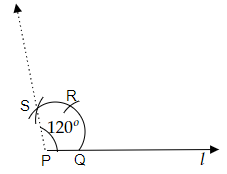Steps of construction:

(i) Let us draw a line $l$ of any length and mark a point $P$ on the line $l$. Now, by taking compasses with any radius let us draw an arc from point $P$ and mark the point of intersection of the arc with the line $l$ as point $Q$.

(ii) Now, by taking compasses with the same radius as before let us draw another arc from point $Q$ and mark the point of intersection of this arc with the previously drawn arc as point $R$.

(iii) Now, by taking the compasses with the same radius as before let us draw another arc from point $R$ and mark the point of intersection of this arc with the first arc and mark the point of intersection as point $S$.

(iv) Now, join the points $S$ and $P$. Therefore, the required angle of measure $120^o$ with the line $l$ is formed.

(e)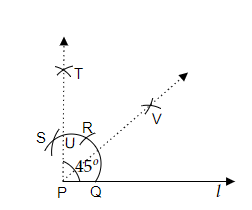Steps of construction:

(i) Let us draw a line $l$ of any length and mark a point $P$ on the line $l$.

(ii) Now, by taking compasses of any radius let us draw an arc from point $P$ and mark the point of intersection of this arc with line $l$ as point $Q$.

(iii) Now, by taking the compasses with the same radius as before let us draw an arc from point $Q$ and mark the point of intersection of this arc with the previous arc as point $R$.

(iv) Now, by taking compasses with the same radius as before let us draw an arc from point $R$ and mark the point of intersection of this arc with the first arc as point $S$.

(v) Now, by taking compasses with the same radius as before let us draw two arcs from point $R$ and point $S$ respectively and mark the point of intersection of these arcs as point $T$. Let us join point $T$ and point $P$.

(vi) $\overline{PT}$ intersects the first drawn arc at some point let us mark this point as point $U$.

(vii) Now, by taking compasses with the measure of radius greater than half of the length from point $Q$ to $U$ let us draw two arcs from point $Q$ and point $U$ respectively and mark the point of intersection of these arcs to each other as $V$.

(viii) Now, let us join point $P$ and point $V$. Therefore, the required angle of measure $45^o$ with the line $l$ is formed.

(f)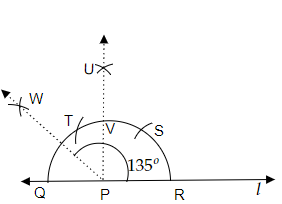Steps of construction:

(i) Let us draw a line $l$ of any length and mark a point on the line $l$.

(ii) Now, by taking compasses of any radius let us draw a semi-circle from point $P$ and mark the point of intersection of this semi-circle with the line $l$ as point $Q$ and point $R$ respectively.

(iii) Now, by taking compasses with the same radius as before let us draw an arc from point $R$ and mark the point of intersection of this arc with the previous semicircle as point $S$.

(iv) Now, by taking compasses with the radius same as before let us draw an arc from point $S$ and mark the point of intersection of this arc with the semicircle as point $T$.

(v) Now, by taking the same radius as before let us draw two arcs from point $S$ and point $T$ respectively and mark the point of intersection of these arcs to each other as point $U$.

(vi) Now, let us join point $P$ and point $U$ and mark the point of intersection of this $\overline{PU}$ to the semicircle as point $v$.

(vii) Now, by taking compasses with a radius greater than half of the length from point $Q$ to point $V$ let us draw an arc and mark the point of intersection of these arcs to each other as point $W$.

(viii) Now, let us join point $P$ and point $W$. therefore, the required angle of measure $135^o$ with the line $l$ is formed.

Updated on: 10-Oct-2022

31 Views